﻿ Coil Spring Attachement on Lathe: Evaluation of the Spring-back EffectFrontiers in Science

p-ISSN: 2166-6083    e-ISSN: 2166-6113

2017;  7(2): 27-30

doi:10.5923/j.fs.20170702.01### Coil Spring Attachement on Lathe: Evaluation of the Spring-back Effect

Olunlade B. A., Joseph S. A., Fatinikun D. E., Fadipe S. K., Bishi M. A., Aliu T. O., Odiba O.

Prototype Engineering Development Institute, National Agency for Science and Engineering Infrastructure, Ilesa, Nigeria

Correspondence to: Odiba O., Prototype Engineering Development Institute, National Agency for Science and Engineering Infrastructure, Ilesa, Nigeria.
 Email: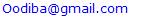Abstract

A coil is created by undergoing a series of transformations from a primary to secondary structure. The primary structure is the “stock” wire, which is fabricated in linear form with a diameter (DW) of any range. The stock wire diameter, DW, is the central factor in determining coil “stiffness”. The stock wire is wound around a mandrel of varying diameter to produce the secondary structure of the coil. The stiffness of a spring is directly proportional to its spring constant (k). When a spring is coiled, its internal diameter does not assume the external diameter of the mandrel; the spring will naturally open to create a diameter larger than the form. This is probably due to the properties of the material and this effect is known as “spring-back effect’’. This paper, therefore, evaluates how varying mandrel sizes affect the air-gap created due to spring-back effect and the possibility of determining the correct mandrel size to be used in obtaining a desired spring size considering tungsten wire.

Keywords: Stock wire, Mandrel, Coil stiffness, Spring-back effect, Spring constant, Secondary structure

Cite this paper: Olunlade B. A., Joseph S. A., Fatinikun D. E., Fadipe S. K., Bishi M. A., Aliu T. O., Odiba O., Coil Spring Attachement on Lathe: Evaluation of the Spring-back Effect, Frontiers in Science, Vol. 7 No. 2, 2017, pp. 27-30. doi: 10.5923/j.fs.20170702.01.

### 1. Introduction

Coil technology has grown substantially with manufacturers releasing different products to the market from time to time. The choice of coil depends on factors such as size, composition, stiffness, and detachment mechanism. The framework of fundamentals of coil designs helps to comprehend the practical nuances of coil development [1, 2].
Metal alloys, such as nitinol, platinum, nickel, iridium, and tungsten are the primary metals used in construction of coils and are developed as alloys for optimal strength . The strength of the wire used in the development of coil depends on its modulus of rigidity or shear modulus (G) which can be experimentally determined from the slope of a stress-strain curve created during torsion tests conducted on a metal sample .
Metal strength is determined experimentally and is referred to as the modulus of rigidity or shear modulus (G). The modulus of rigidity is the coefficient of elasticity for a shearing force, defined as the ratio of the shear stress to the shear strain .
A coil is created by undergoing a series of transformations from a primary (1°) to secondary (2°). The primary structure is the “stock” wire, which is fabricated in linear form with a diameter (DW) of any range. The stockwire diameter, DW, is the central factor in determining coil “stiffness” . The stock wire is wound around a mandrel of varying diameter to produce the secondary structure of the coil. To understand coil stiffness, one needs to reference the physical properties of springs. The stiffness of a spring is directly proportional to its spring constant (k) [5, 6].
When a spring is coiled, its internal diameter does not assume the external diameter of the mandrel; the spring will naturally open to create a diameter larger than the form. This is probably due to the properties of the material and this is known as ‘’ spring-back effect’’ . Hence, the objective of this paper is to evaluate the significance of the spring-back effect on varying sizes of mandrel for spring coiling.

### 2. Methodology

The methodology adopted in this paper includes:

#### 2.1. Definition of Variables

The factors considered in spring making are; the type of wire to use, the size of the wire, number of coils, length of spring and size of mandrel. Since the work is about spring-back effect on transformation from primary to secondary structure, the number of coils and length of spring are not necessary factors to be considered.
The internal diameter of the coiled spring is the sum of the desired diameter of the spring and the air-gap created as a result of the spring-back effect , that is;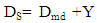(1)
where;
DS is the inner diameter of the spring after coiling,
Dmd is the desired diameter of the spring,
Y is the air-gap created due to spring back effect,
Upon release of applied stress, a wire rod is bent around a mandrel of a given radius of curvature larger than the mandrel radius. For round wire or rod subject to bending around a mandrel of radius R0, the spring-back is described by the formula,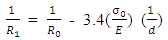(2)
where R1 is the radius of curvature of the wire after spring-back .
Hence, from equation 2, the radius of the spring formed after coiling is,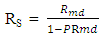(3)
where,
RS = R1 is the radius of spring after coiling,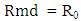is the radius of the mandrel used in making the spring,
In this paper, C is given as the spring constant, as obtained from equation 4;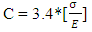(4)
The wire constant, C, includes the mechanical properties of the material used which depends on the Young Modulus, E and the flow stress σ, of the material used in making the spring . The material considered in this paper is tungsten.
The actual size of mandrel needed to produce a spring of a desired diameter is obtained from,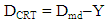(5)

#### 2.2. Computation of Variables

The variables were computed on spreadsheet shown in figure 1 to generate values for the desired spring diameter (Dmd) and the air gap created due to spring-back effect (Y).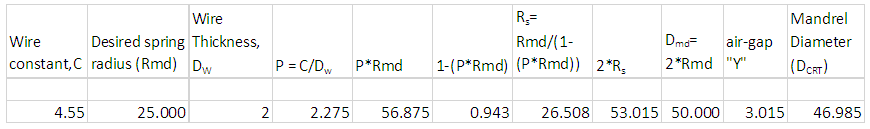Figure 1. Spreadsheet for generating data for determining the relationship between “Air-gap, Y” and “Desired Spring Diameter, Dmd for varying wire thickness (Dw) of tungsten

### 3. Result and Discussion

Graphs (figures 2-7) were plotted for different thickness to observe the extent to which increase in mandrel diameter affects the air-gap created due to spring-back effect considering tungsten wire.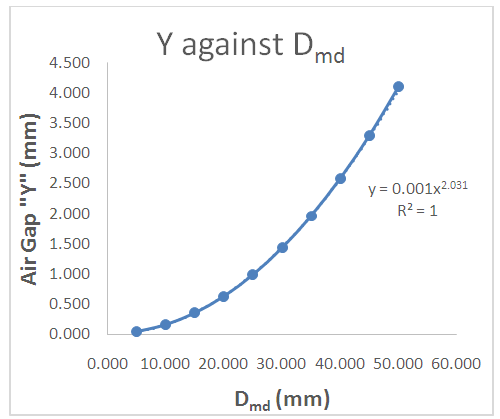Figure 2. Plot of Air Gap “Y’ against Desired Spring Diameter “Dmd” for tungsten wire of 1.5mm thickness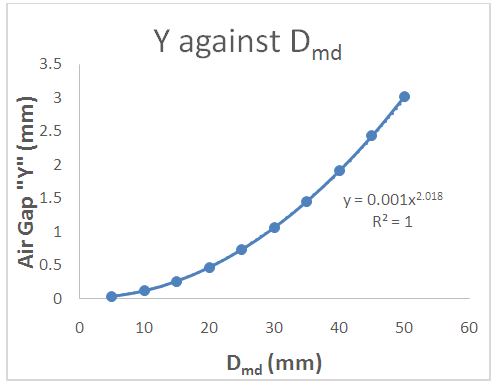Figure 3. Plot of Air Gap “Y’ against Desired Spring Diameter “Dmd” for tungsten wire of 2.0mm thickness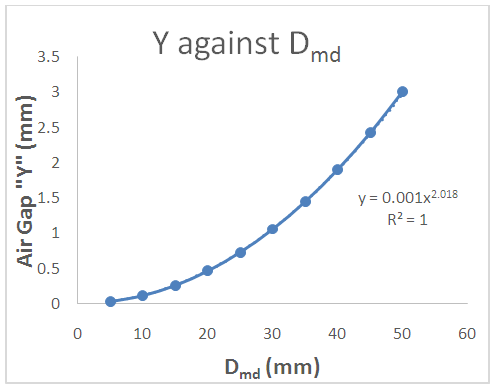Figure 4. Plot of Air Gap “Y’ against Desired Spring Diameter “Dmd” for tungsten wire of 2.0mm thickness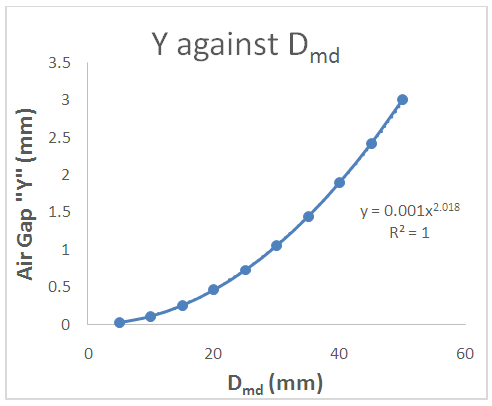Figure 5. Plot of Air Gap “Y” against Desired Spring Diameter “Dmd” for tungsten wire of 3.0mm thickness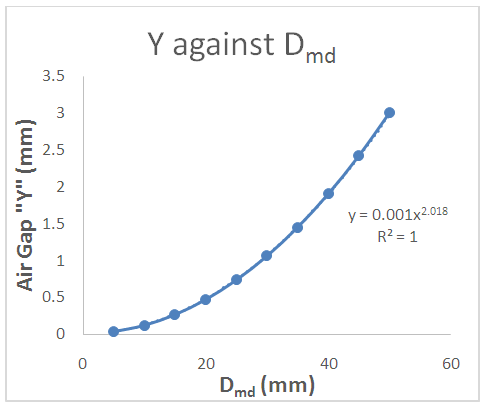Figure 6. Plot of Air Gap “Y” against Desired Spring Diameter “Dmd” for tungsten wire of 3.5mm thickness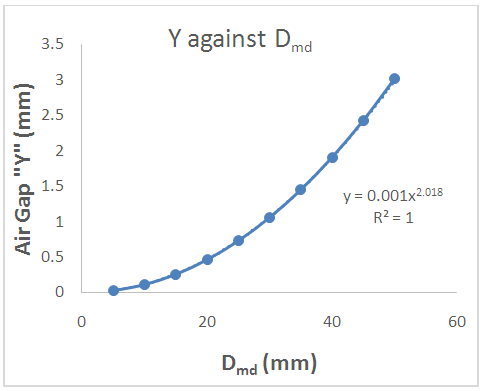Figure 7. Plot of Air Gap “Y” against Desired Spring Diameter “Dmd” for tungsten wire of 4.0mm thickness
The following are deduced from the graph:
ü The air-gap between the coil and the mandrel increases with increase in diameter of the mandrel used in making the spring as indicated by each graph.
ü The value of correlation coefficient, R2, obtained from the plot indicates that there is a strong relationship between the mandrel size and the air-gap created due to spring back effect i.e. the air-gap is dependent on the mandrel diameter as indicated by the R2 of each graph.
ü The model to obtain the values of Y is generated from the graphs.
ü Comparing figures 2-7, the higher the thickness of the wire, the more linear the plot.

### 4. Conclusions

Conclusively, the work has shown dependence of air-gap on the size of the mandrel, that is the higher the mandrel diameter, the higher the value of the air-gap. Hence, the diameter of the mandrel to be used in making a spring of a particular diameter can be determined by subtracting the value of the air-gap obtained from the desired diameter of a spring. Also, the spreadsheet caters for tungsten wire of thickness less than 10mm, hence if the desired radius and thickness are known, the mandrel size to be used can be generated from the sheet. Also, from figures 2-7 we can conclude that tungsten material behaves the same way irrespective of thickness when used for spring.

### References

  Wahl, A. M. (1944). Mechanical springs. Machine Design Series, 1st Edition, Penton Publishing co. Ohio, U.S.A.  Prawoto, Y., Ikeda. M., Manville, .S. K., Nishikawa, A. (2008). Design and Failure Modes of Automotive Suspension Springs. Engineering Failure Analysis. 15(8):1155-1174.  Roark, R. J. (1965). Formulas for Stress and Strain, 4th Edition, McGraw-Hill, New York.  Valsange, P. S. (2012). Design of Helical Coil Compression Spring “A Review”. International Journal of Engineering Research and Applications. 2(6):513-522.  White, J. B., Ken, C. G. M., Cloft, H. J. and Kallmes, D. F. (2008). Coils in a Nutshell: A Review of Coil Physical Properties. American Society of Neuroradiology.pp 1-5.  Timoshenko, S.P. and Goodier, J.N. (1970). Theory of Elasticity, McGraw-Hill, New York.  Eric Kam (2007). Clearing the springback hur dle. Stamping Journal, Sept. 2007  Khurmi, R. S. and Gupta, J. K. (2010). Machine Design, 14th Edition, S. Chand, New Delhi, India.  Erik Lassner and Wolf-Dieter Schubert (1999). Tungsten: Properties, Chemistry, Technology of Elements, Alloys and Chemical Compounds. Publisher: Springer.  Wright, R. N. (2010). Wire Technology: Process Technology and Metallurgy, 2nd Edition, Elsevier Store, USA.